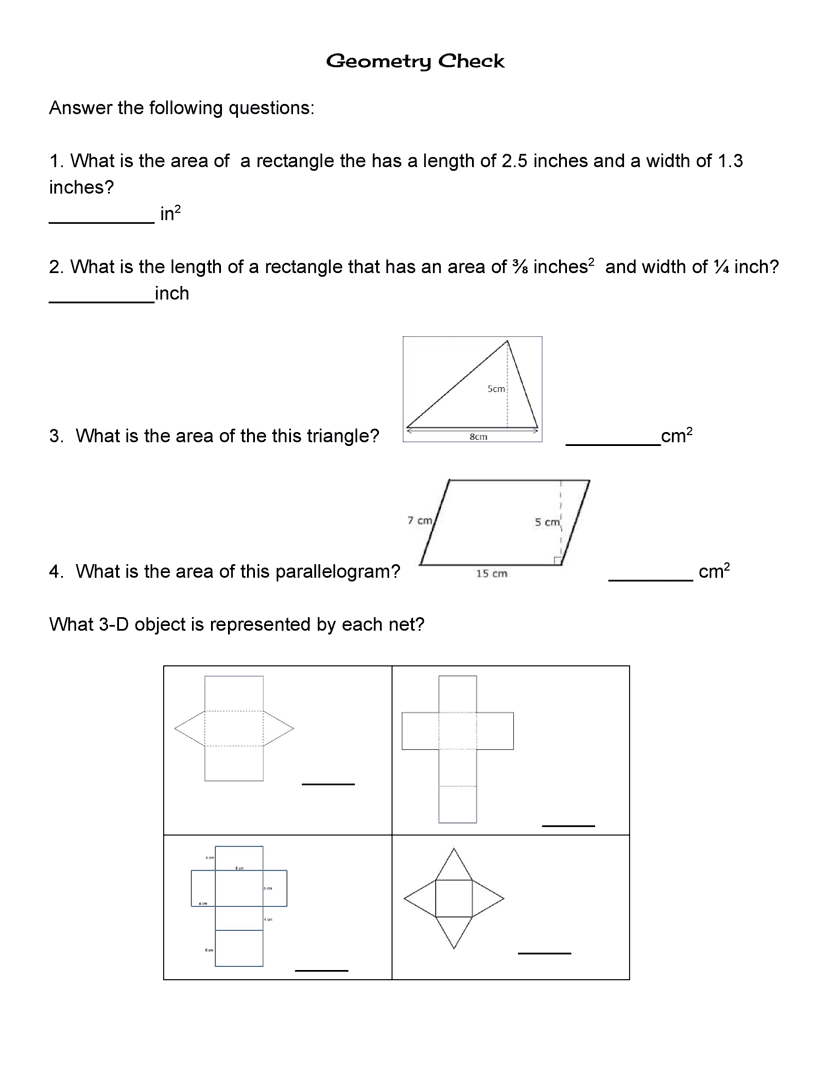Geometry Check
starstarstarstarstarstarstarstarstarstar
by Polly Leasure
| 12 Questions1
2
3
4
5
6
7
8
1
1 pt
2
1 pt
Type answer as a improper fraction.
3
1 pt
4
1 pt
5
1 pt
Triangular pyramid
Rectangular pyramid
Triangular prism
Rectangular prism
6
1 pt

rectangular pyramid
cube
rectangular prism
square
7
1 pt

rectangular prism
rectangular pyramid
cube
triangular prism
8
1 pt

triangular pyramid
rectangular pyramid
square pyramid
square prism9
10
11
12
9
1 pt

pentagonal prism
hexagonal pyramid
octagonal prism
hexagonal prism
10
1 pt

triangular prism
triangular pyramid
square pyramid
rectangular pyramid
11
1 pt

hexagonal pyramid
octagonal pyramid
pentagonal pyramid
pentagonal prism
12
1 pt

hexagonal prism
octagonal prism
pentagonal prims
rectangular prism